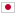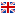B - Colorful Drink/### Problem Statement

In the Jambo Amusement Garden (JAG), you sell colorful drinks consisting of multiple color layers. This colorful drink can be made by pouring multiple colored liquids of different density from the bottom in order.

You have already prepared several colored liquids with various colors and densities. You will receive a drink request with specified color layers. The colorful drink that you will serve must satisfy the following conditions.

• You cannot use a mixed colored liquid as a layer. Thus, for instance, you cannot create a new liquid with a new color by mixing two or more different colored liquids, nor create a liquid with a density between two or more liquids with the same color by mixing them.
• Only a colored liquid with strictly less density can be an upper layer of a denser colored liquid in a drink. That is, you can put a layer of a colored liquid with density $x$ directly above the layer of a colored liquid with density $y$ if $x < y$ holds.

Your task is to create a program to determine whether a given request can be fulfilled with the prepared colored liquids under the above conditions or not.

### Input

The input consists of a single test case in the format below.

$N$ $C_1$ $D_1$ $\vdots$ $C_N$ $D_N$ $M$ $O_1$ $\vdots$ $O_M$

The first line consists of an integer $N$ ($1 \le N \le 10^5$), which represents the number of the prepared colored liquids. The following $N$ lines consists of $C_i$ and $D_i$ ($1 \leq i \leq N$). $C_i$ is a string consisting of lowercase alphabets and denotes the color of the $i$-th prepared colored liquid. The length of $C_i$ is between $1$ and $20$ inclusive. $D_i$ is an integer and represents the density of the $i$-th prepared colored liquid. The value of $D_i$ is between $1$ and $10^5$ inclusive. The ($N+2$)-nd line consists of an integer $M$ ($1 \leq M \leq 10^5$), which represents the number of color layers of a drink request. The following $M$ lines consists of $O_i$ ($1 \leq i \leq M$). $O_i$ is a string consisting of lowercase alphabets and denotes the color of the $i$-th layer from the top of the drink request. The length of $O_i$ is between $1$ and $20$ inclusive.

### Output

If the requested colorful drink can be served by using some of the prepared colored liquids, print Yes. Otherwise, print No.

### Sample Input 1

2
white 20
black 10
2
black
white


### Output for Sample Input 1

Yes


### Sample Input 2

2
white 10
black 10
2
black
white


### Output for Sample Input 2

No


### Sample Input 3

2
white 20
black 10
2
black
orange


### Output for Sample Input 3

No


### Sample Input 4

3
white 10
red 20
white 30
3
white
red
white


### Output for Sample Input 4

Yes


### Sample Input 5

4
red 3444
red 3018
red 3098
red 3319
4
red
red
red
red


### Output for Sample Input 5

Yes